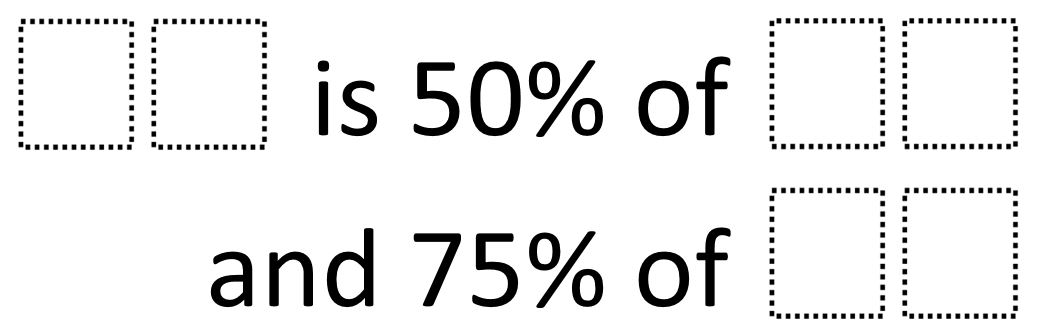Home > Grade 6 > Related Percentages

# Related Percentages

Directions: Using the digits 0 to 9 as many times as you want, fill in the boxes to create a correct number sentence.### Hint

This problem could also be stated, “x is 50% of y and 75% of z”. Start by considering x is 50% of y. What numbers satisfy this relationship? What do you notice about these numbers. Now consider that x is also 75% of z. What do you know about numbers that must be 1/2 of a number and also 3/4 of a number?

There are 17 different solutions to this question. A few of these are: 6 is 50% of 12 and 75% of 8
15 is 50% of 30 and 75% of 20
21 is 50% of 42 and 75% of 28
42 is 50% of 84 and 75% of 56
There are 4 different solutions to the challenge question:
18 is 50% of 36 and 75% of 24
27 is 50% of 54 and 75% of 36
36 is 50% of 72 and 75% of 48
39 is 50% of 78 and 75% of 52

Extension/Challenge: Try to create this number sentence using each digit no more than once.

Source: Erick Lee

## Prime Factorization 2

Directions: Using the digits 0 to 9 at most one time each, fill in the …

1.30 50% 60 and 75% 40

2.06 is 50% of 12 and is 75% of 8

3.03 is 50% of 06 and is 75% of 4.

4.09 is 50% of 18 and 75% of 12
12 is 50% 24 and is 75% of 16
15 is 50% of 30 and 75% of 20
18 is 50% of 36 and 75% of 24
21 is 50% of 42 and 75% of 28
24 is 50% of 48 and 75% of 32
27 is 50% of 54 and 75% of 36
30 is 50% of 60 and 75% of 40
33 is 50% of 66 and is 75% 44
36 is 50% of 72 and is 75% of 48
39 is 50% of 78 and is 75% of 56
42 is 50% of 84 and is 75% of 60
45 is 50% of 90 and is 75% of 64
48 is 50% of 96 and is 75% of 68

5.30 50% 60 and 75% 40

6.21 is 50% of 42 and 75% of 28

7.15 is 50% of 30 and 75% of 20